Atmos. Meas. Tech., 15, 987–1006, 2022
https://doi.org/10.5194/amt-15-987-2022
Atmos. Meas. Tech., 15, 987–1006, 2022
https://doi.org/10.5194/amt-15-987-2022

Research article 24 Feb 2022

Research article | 24 Feb 2022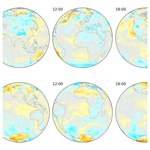# Calculating the vertical column density of O4 during daytime from surface values of pressure, temperature, and relative humidity

Calculating the vertical column density of O4 during daytime from surface values of pressure, temperature, and relative humidity
Steffen Beirle, Christian Borger, Steffen Dörner, Vinod Kumar, and Thomas Wagner Steffen Beirle et al.
• Satellitenfernerkundung, Max-Planck-Institut für Chemie, Mainz, Germany

Correspondence: Steffen Beirle (steffen.beirle@mpic.de)

Abstract

We present a formalism that relates the vertical column density (VCD) of the oxygen collision complex O2O2 (denoted as O4 below) to surface (2 m) values of temperature and pressure, based on physical laws. In addition, we propose an empirical modification which also accounts for surface relative humidity (RH). This allows for simple and quick calculation of the O4 VCD without the need for constructing full vertical profiles. The parameterization reproduces the true O4 VCD, as derived from vertically integrated profiles, within $-\mathrm{0.7}±\mathrm{1.2}\phantom{\rule{0.125em}{0ex}}\mathit{%}$ (mean ± SD) for Weather Research and Forecasting (WRF) simulations around Germany, 0.2±1.8 % for global reanalysis data (ERA5), and $-\mathrm{0.3}±\mathrm{1.4}\phantom{\rule{0.125em}{0ex}}\mathit{%}$ for Global Climate Observing System (GCOS) Reference Upper-Air Network (GRUAN) radiosonde measurements around the world. When applied to measured surface values, uncertainties of 1 K, 1 hPa, and 16 % for temperature, pressure, and RH correspond to relative uncertainties of the O4 VCD of 0.3 %, 0.2 %, and 1 %, respectively. The proposed parameterization thus provides a simple and accurate formula for the calculation of the O4 VCD which is expected to be useful in particular for MAX-DOAS applications.

Share
1 Introduction

In the atmosphere, two oxygen molecules can build collision pairs and dimers, which are often denoted as O4 (, and references therein). O4 has absorption bands in the UV–visible spectral range; thus O4 can be retrieved from atmospheric absorption spectra, e.g., by applying differential optical absorption spectroscopy (DOAS) . Measurements of the O4 absorption pattern in scattered light provide information about light path distributions in the atmosphere, for instance allowing the investigation of light path length increase within clouds or the retrieval of cloud heights from satellite measurements .

For multi-axis (MAX) DOAS, i.e., ground-based instruments measuring scattered light at different elevation angles, O4 measurements provide information on vertical profiles of aerosol extinction . Prerequisite for MAX-DOAS profile inversions is knowledge about the O4 vertical column density (VCD) which provides the link between the measured slant column densities (SCDs) at different viewing angles and the forward modeled SCDs based on radiative transfer calculations. Thus, a wrong input of the O4 VCD directly affects the resulting aerosol profiles. For the profile inversion algorithm MAPA applied to measurements taken during the CINDI-2 campaign , for instance, a change of the input O4 VCD of 2 %, 3 %, 5 %, or 10 % causes changes of the resulting median aerosol optical depth of 6 %, 8 %, 13 %, or 20 %, respectively. Thus, for MAX-DOAS profile inversions, the O4 VCD should be determined with accuracy and precision better than about 3 %, which limits the impact on resulting aerosol optical depths (AODs) to below 10 % and leaves other sources of uncertainty, i.e., the spectral analysis (≈5 %) as well as radiative transfer modeling (≈4 %) (see ; Table 3 therein) as the limiting factors.

The O4 VCD can be calculated by vertical integration of the O2 number density profile squared. This requires knowledge of vertical profiles of temperature, pressure, and humidity, e.g., as derived from radiosonde measurements or meteorological models. However, radiosonde measurements are only available for few stations and do not provide continuous temporal coverage, while modeled profiles might not be available in some cases (e.g., during measurement campaigns in remote regions and poor internet connection; for these cases, profiles from a climatology might be used as fallback option), or they might not reflect the conditions at the measurement site appropriately, in particular in mountainous terrain not resolved by the model.

Measurements of surface air (at 2 m) temperature, pressure, and humidity, on the other hand, are routinely performed by meteorological stations and could be added to any MAX-DOAS measurement site with relatively low costs and efforts. proposed to construct full temperature and pressure profiles from the respective surface values by assuming (a) a constant lapse rate of −6.5 K km−1 from ground up to 12 km, and constant temperature above, and (b) applying the barometric formula.1 estimate the uncertainty of the calculated O4 VCD to 3 % and list the diurnal variation of the surface temperature and the limited representativeness of the surface temperature for the temperature profile above the boundary layer as the main source of uncertainty.

The method proposed by reproduces the true O4 VCD within about 2 % (bias) ±2 % standard deviation (SD) globally when compared to ECMWF profiles. Locally, however, large deviations up to 7 % could be found, as shown in the current study, which is mainly caused by the assumption of a fixed lapse rate of −6.5 K km−1: while this value reflects typical continental conditions quite well, it is not appropriate in particular over deserts, where lapse rates are stronger (closer to the dry adiabatic lapse rate), and parts of the oceans with weaker (i.e., closer to zero) lapse rates due to condensation.

In this paper we present a simpler approach for the calculation of O4 VCD just from surface values of temperature and pressure and an a priori lapse rate based on physical laws, without the need of constructing full profiles. In addition, we provide an empirical parameterization involving surface relative humidity that also accounts for variations of the atmospheric lapse rate. This allows for simple and quick calculation of the O4 VCD with high accuracy and precision just from surface measurements of temperature, pressure, and relative humidity.

The article derives the formalism of the parameterizations of the O4 VCD in Sect. 2. In Sect. 3, the datasets used for illustration and quantification of uncertainties are introduced, followed by applications of the O4 parameterizations in Sect. 4. Important aspects like comparison to standard methods used for the calculation of the O4 VCD, the impacts of temperature inversions, surface altitude, or diurnal cycles, and the accuracy and precision of the proposed parameterizations are discussed in Sect. 5, followed by conclusions.

2 Formalism

In this section, we provide the formalism for the calculation of O4 VCDs from surface values of pressure, temperature, and relative humidity.

## 2.1 Notation

Basic quantities of the derivation below are (a) the number density n and (b) the vertical column density (VCD) V, i.e., the vertically integrated number density.

The O4 number density is just defined as the O2 number density squared. Consequently, the O4 number density has the unit molecules2 cm−6, and the O4 VCD has the unit molecules2 cm−5. This matches the common procedure in the DOAS community; the O4 cross section is given in cm5 molecules−2 accordingly .

Pressure is denoted by p, temperature by T, and the altitude above sea level by z, while altitude above ground level is denoted by z. For relative humidity, RH is used in the text as well as in formulas. Surface values are indicated by the subscript “0”. Quantities related to O2 and O4 are indicated by a respective subscript. For a full list of quantities and symbols see Tables 1 and 2.

Table 1Variables used in this study. A subscript of 0 indicates surface values for n, p, T, z, or RH.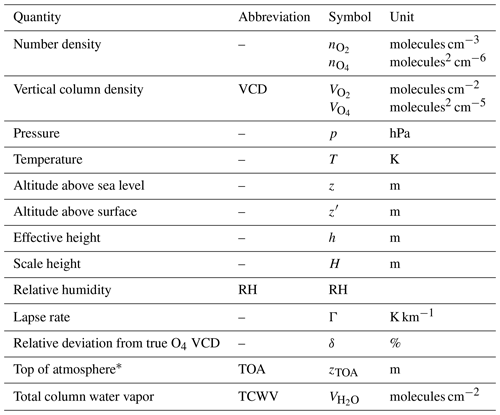* Here: highest available/possible profile layer.

Table 2Constants used in this study. Numbers are listed with six digits.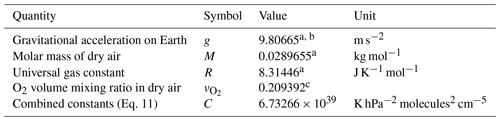a From the Python module MetPy .
b Latitudinal variations of g are below ±0.27 % and are neglected in this study.
c From .

## 2.2 General approach

The VCD V is the vertically integrated number density n:

$\begin{array}{}\text{(1)}& V=\underset{{z}_{\mathrm{0}}}{\overset{\mathrm{\infty }}{\int }}n\left(z\right)\phantom{\rule{0.25em}{0ex}}\mathrm{d}z\phantom{\rule{0.125em}{0ex}}.\end{array}$

This integral can be re-written as

$\begin{array}{}\text{(2)}& V={n}_{\mathrm{0}}\cdot h,\end{array}$

with

$\begin{array}{}\text{(3)}& h=\underset{{z}_{\mathrm{0}}}{\overset{\mathrm{\infty }}{\int }}\frac{n\left(z\right)}{{n}_{\mathrm{0}}}\phantom{\rule{0.25em}{0ex}}\mathrm{d}z.\end{array}$

This effective height h can be understood as the height of the gas column if the gas would be in a homogenous box under surface conditions p0 and T0. Note that the effective height equals the scale height H only in the case of exponential profiles, i.e., an isothermal atmosphere (see Appendix A).

Thus, the VCDs for O2 and O4 can be written as

$\begin{array}{}\text{(4)}& {V}_{{\text{O}}_{\mathrm{2}}}={n}_{{\text{O}}_{\mathrm{2}},\mathrm{0}}\cdot {h}_{{\text{O}}_{\mathrm{2}}},\end{array}$

and

$\begin{array}{}\text{(5)}& {V}_{{\text{O}}_{\mathrm{4}}}={n}_{{\text{O}}_{\mathrm{4}},\mathrm{0}}\cdot {h}_{{\text{O}}_{\mathrm{4}}}={n}_{{\text{O}}_{\mathrm{2}},\mathrm{0}}^{\mathrm{2}}\cdot {h}_{{\text{O}}_{\mathrm{4}}}.\end{array}$

Re-arranging Eq. (4) for ${n}_{{\text{O}}_{\mathrm{2}},\mathrm{0}}$ and replacing one ${n}_{{\text{O}}_{\mathrm{2}},\mathrm{0}}$ term in Eq. (5) yields

$\begin{array}{}\text{(6)}& {V}_{{\text{O}}_{\mathrm{4}}}={V}_{{\text{O}}_{\mathrm{2}}}\cdot {n}_{{\text{O}}_{\mathrm{2}},\mathrm{0}}\cdot \frac{{h}_{{\text{O}}_{\mathrm{4}}}}{{h}_{{\text{O}}_{\mathrm{2}}}}.\end{array}$

Hence the O4 VCD can be expressed as the product of the O2 VCD, the O2 surface number density, and the ratio of effective heights of O2 and O4 profiles. So far, no simplifications or approximations have been made; thus Eq. (6) holds for any atmospheric conditions.

## 2.3O4 VCD as a function of surface pressure, surface temperature, and lapse rate

Based on Eq. (6), the O4 VCD can be related to surface pressure, surface temperature, and lapse rate, if some further assumptions are made:

1. Assuming a hydrostatic atmosphere, the surface pressure is just the gravitational force per area of the total air column. Thus, the O2 VCD is directly related to the surface pressure:

$\begin{array}{}\text{(7)}& {V}_{{\text{O}}_{\mathrm{2}}}=\frac{{\mathit{\nu }}_{{\text{O}}_{\mathrm{2}}}}{g\cdot M}\cdot {p}_{\mathrm{0}},\end{array}$

with ${\mathit{\nu }}_{{\text{O}}_{\mathrm{2}}}$ being the volume mixing ratio of O2 in dry air, g being the gravitational acceleration on Earth, and M being the molar mass of dry air.

2. According to the ideal gas law for dry air, the surface number density of O2 can be expressed as

$\begin{array}{}\text{(8)}& {n}_{{\text{O}}_{\mathrm{2}},\mathrm{0}}=\frac{{\mathit{\nu }}_{{\text{O}}_{\mathrm{2}}}}{R}\cdot \frac{{p}_{\mathrm{0}}}{{T}_{\mathrm{0}}},\end{array}$

with the universal gas constant R.

3. The ratio of effective heights for O2 and O4 depends on the actual profile shape for O2. For some specific cases, the integral in Eq. (3) can be solved analytically (see Appendix A for details):

• For an isothermal atmosphere, i.e., an exponential profile of ${n}_{{\text{O}}_{\mathrm{2}}}$, the ratio $\frac{{h}_{{\text{O}}_{\mathrm{2}}}}{{h}_{{\text{O}}_{\mathrm{4}}}}$ is just 2 (Eq. A2).

• For a constant lapse rate Γ, the ratio becomes $\mathrm{2}+\frac{R}{g\cdot M}\mathrm{\Gamma }$ (Eq. A10).

• For real atmospheric conditions, where the lapse rate varies with altitude, the ratio of effective heights can be still described by Eq. (A10) if an effective lapse rate is considered:

$\begin{array}{}\text{(9)}& {\mathrm{\Gamma }}_{\text{eff}}:=\left(\frac{{h}_{{\text{O}}_{\mathrm{2}}}}{{h}_{{\text{O}}_{\mathrm{4}}}}-\mathrm{2}\right)\cdot \frac{g\cdot M}{R}.\end{array}$

For a given atmospheric profile, the effective lapse rate is thus defined as the lapse rate of a polytropic atmosphere of the same O4 VCD.

Replacing the terms in Eq. (6) by Eqs. (7), (8) and (A10) yields

$\begin{array}{}\text{(10)}& \begin{array}{rl}{V}_{{\text{O}}_{\mathrm{4}},\mathrm{\Gamma }}& =\frac{{\mathit{\nu }}_{{\text{O}}_{\mathrm{2}}}^{\mathrm{2}}}{R\cdot g\cdot M}/\left(\mathrm{2}+\frac{R}{g\cdot M}\mathrm{\Gamma }\right)\cdot \frac{{p}_{\mathrm{0}}^{\mathrm{2}}}{{T}_{\mathrm{0}}}\\ & =\frac{C}{\mathrm{2}+\frac{R}{g\cdot M}\mathrm{\Gamma }}\cdot \frac{{p}_{\mathrm{0}}^{\mathrm{2}}}{{T}_{\mathrm{0}}}\end{array}\end{array}$

with

$\begin{array}{}\text{(11)}& C=\frac{{\mathit{\nu }}_{{\text{O}}_{\mathrm{2}}}^{\mathrm{2}}}{R\cdot g\cdot M}\end{array}$

combining the constant factors. Thus with the assumptions specified above, the O4 VCD is proportional to ${p}_{\mathrm{0}}^{\mathrm{2}}/{T}_{\mathrm{0}}$, with the lapse rate Γ determining the slope.

## 2.4 Calculation of the “true” O4 VCD

In order to evaluate the performance of the parameterizations for the O4 VCD, we also calculate the “true” O4 VCD, which is derived by (a) calculating the profile of ${n}_{{\text{O}}_{\mathrm{2}}}$ from profiles of T, p, and RH. In this step, the effect of humidity is explicitly accounted for by subtracting the water vapor pressure before calculating ${n}_{{\text{O}}_{\mathrm{2}}}$ based on the ideal gas law, (b) performing the numerical integration (using Simpson's rule) of ${n}_{{\text{O}}_{\mathrm{2}}}^{\mathrm{2}}$ from surface to top of atmosphere (TOA).

The integration has to be performed up to sufficiently high altitudes ( recommend zTOA≥30 km) as otherwise the integrated VCD would be biased low due to the missing column above. As not all datasets considered below cover this altitude range, we estimate and correct for the missing O4 column above the highest profile level by applying Eq. (10) for the highest available layer, assuming a lapse rate of zero above. Note that the temperature increase in the upper stratosphere is not relevant here as the contribution to the O4 VCD above 30 km is negligible. Thus, the “true” O4 VCD is calculated as

$\begin{array}{}\text{(12)}& {V}_{{\text{O}}_{\mathrm{4}},\text{true}}=\underset{{z}_{\mathrm{0}}}{\overset{{z}_{\text{TOA}}}{\int }}{n}_{{\text{O}}_{\mathrm{2}}}^{\mathrm{2}}\left(z\right)\mathrm{d}z+\frac{C}{\mathrm{2}}\cdot \frac{{p}_{\text{TOA}}^{\mathrm{2}}}{{T}_{\text{TOA}}}.\end{array}$

For zTOA of 20 km, the correction term is of the order of 0.3 % of the total O4 column.

## 2.5O4 VCD as a function of surface pressure, surface temperature, and surface relative humidity

Equation (10) might be applied for a common lapse rate, like −6.5 K km−1 as proposed in . This works generally well over most continental regions. However, large deviations have to be expected for regions with different lapse rates, in particular over deserts, where lapse rates are typically stronger (more negative, i.e., close to the dry adiabatic lapse rate). Over parts of the ocean, on the other hand, lapse rates are weaker (closer to zero).

In order to modify Eq. (10) such that it can be applied globally, but keep it still a simple function of surface measurements, we make use of the relation between the effective lapse rate and the RH at ground:

• For ascending air masses, RH0 determines the altitude at which condensation takes place. This relation is directly reflected in the calculation of the lifted condensation level (LCL) as function of RH0 . Thus, the lower RH0, the higher the altitude range above ground where dry adiabatic lapse rates apply.

• For descending air masses (in particular the large-scale subsidence over tropical deserts), no condensation takes place and dry adiabatic lapse rates apply.

In both cases, low relative humidity at ground is associated with lapse rates closer to the dry adiabatic lapse rate.

Real atmospheric profiles are of course more complex than these simplified scenarios, in particular due to advection, but still, a correlation between RH0 and effective lapse rates is expected. We thus parameterize the effective lapse rate by the relative humidity at ground via a linear function:

$\begin{array}{}\text{(13)}& {\mathrm{\Gamma }}_{\text{eff}}=\mathit{\alpha }+\mathit{\beta }\cdot {\text{RH}}_{\mathrm{0}}.\end{array}$

Replacing this in Eq. (10) results in ${V}_{{\text{O}}_{\mathrm{4}}}$ becoming a function of surface values for pressure, temperature, and relative humidity:

$\begin{array}{}\text{(14)}& \begin{array}{rl}{V}_{{\text{O}}_{\mathrm{4}},\text{RH}}& =\frac{C}{\mathrm{2}+\frac{R}{g\cdot M}\cdot \left(\mathit{\alpha }+\mathit{\beta }\cdot {\text{RH}}_{\mathrm{0}}\right)}\cdot \frac{{p}_{\mathrm{0}}^{\mathrm{2}}}{{T}_{\mathrm{0}}}\\ & =\frac{C}{a+b\cdot {\text{RH}}_{\mathrm{0}}}\cdot \frac{{p}_{\mathrm{0}}^{\mathrm{2}}}{{T}_{\mathrm{0}}},\end{array}\end{array}$

where the parameters a and b are linked to α and β from Eq. (13) via

$\begin{array}{}\text{(15)}& \mathit{\alpha }=\left(a-\mathrm{2}\right)\cdot \frac{g\cdot M}{R}\end{array}$

and

$\begin{array}{}\text{(16)}& \mathit{\beta }=\frac{g\cdot M}{R}\cdot b.\end{array}$

The parameters a and b can then be determined by a linear least squares fit by comparing ${V}_{{\text{O}}_{\mathrm{4}},\text{RH}}$ to ${V}_{{\text{O}}_{\mathrm{4}},\text{true}}$:

$\begin{array}{}\text{(17)}& {V}_{{\text{O}}_{\mathrm{4}},\text{RH}}=\frac{C}{a+b\cdot {\text{RH}}_{\mathrm{0}}}\cdot \frac{{p}_{\mathrm{0}}^{\mathrm{2}}}{{T}_{\mathrm{0}}}\stackrel{!}{=}{V}_{{\text{O}}_{\mathrm{4}},\text{true}};\end{array}$

thus

$\begin{array}{}\text{(18)}& a+b\cdot {\text{RH}}_{\mathrm{0}}\stackrel{!}{=}\frac{C}{{V}_{{\text{O}}_{\mathrm{4}},\text{true}}}\cdot \frac{{p}_{\mathrm{0}}^{\mathrm{2}}}{{T}_{\mathrm{0}}}=:Q.\end{array}$

a and b will be derived in Sect. 4.2 based on true O4 VCDs calculated from ECMWF profiles. This empirical approach also, at least partly, corrects for effects neglected in the derivation of Eq. (10), i.e., ignoring the tropopause in the calculation of the ratio of effective heights (Appendix A3), and applying the ideal gas law for dry air in Sect. 2.3.

From a and b, also the corresponding effective lapse rate for a given RH0 can then be calculated with Eqs. (13), (15), and (16). This lapse rate allows one to construct full atmospheric profiles of T and p (applying the barometric formula for polytropic atmosphere) from surface measurements when needed, in particular for MAX-DOAS inversions based on optimal estimation. As humidity effects are already accounted for in the determination of a and b, no further correction for humidity should be applied in this case.

## 2.6 Comparison of parameterized to “true” O4 VCD

In order to assess accuracy and precision of the proposed calculation of the O4 VCD from surface measurements of T0, p0, and Γ (Eq. 10) or RH0 (Eq. 14), we define the relative deviation δ of a derived O4 VCDs to the true value:

$\begin{array}{}\text{(19)}& {\mathit{\delta }}_{x}=\frac{{V}_{{\text{O}}_{\mathrm{4}},x}-{V}_{{\text{O}}_{\mathrm{4}},\text{true}}}{{V}_{{\text{O}}_{\mathrm{4}},\text{true}}}.\end{array}$

The index x indicates the O4 VCD dataset and is “Γ” for ${V}_{{\text{O}}_{\mathrm{4}},\mathrm{\Gamma }}$ and “RH” for ${V}_{{\text{O}}_{\mathrm{4}},\text{RH}}$.

3 Datasets

For illustration as well as for the quantification of uncertainties, we apply the derived formalism to different atmospheric datasets:

1. global model data, in order to check for the performance of the parameterizations globally, covering the full range of the relevant parameter space for surface values of pressure, temperature, humidity, and altitude;

2. regional model data with high spatial resolution, which are also compared to surface stations and allow the investigation of the impact of diurnal cycles;

3. balloon-borne radiosonde measurements, in order to apply the formalism to high-resolved profile measurements.

Nighttime profiles of T can be considerably different from daytime profiles, in particular in the case of temperature inversions (i.e., positive lapse rates) often occurring within the nocturnal boundary layer. For MAX-DOAS measurements, however, nighttime profiles are irrelevant. Thus, we consider all atmospheric datasets for daytime conditions only. This is done by selecting data with an solar zenith angle (SZA) below 85.

## 3.1 Global model (ECMWF)

We use global model data as provided by the European Centre for Medium-Range Weather Forecasts (ECMWF) for two purposes:

• In order to investigate global patterns, we use ERA5 reanalysis data truncated at wavenumber 639 on the Gaussian grid N320, corresponding to ≈0.3 resolution. Model output is provided hourly. Here, we focus on ERA5 data for four selected days, i.e., 18 March, 18 June, 18 September, and 18 December 2018, covering the full globe for all seasons. As the regular latitude–longitude grid over-represents high latitudes, we only consider the fraction of cos(lat) pixels for each latitude for the calculation of histograms, correlation coefficients, means, and standard deviations.

• For comparison with the standard approach for the calculation of the O4 VCD that was used in MAPA so far, we use ERA-Interim reanalysis data truncated at wavenumber 255, corresponding to ≈0.7 resolution, which was preprocessed to a dataset with 6 hourly model output (00:00, 06:00, 12:00, 18:00 UTC) interpolated to a regular horizontal grid with a resolution of 1. This dataset is denoted as ERA_Idaily below.

In addition, we make use of a monthly climatology of atmospheric profiles (ERA_Iclim) based on the same ERA-Interim data, which was constructed as back-up solution recommended within the FRM4DOAS project in case no other profile information is available.

## 3.2 Regional model (WRF-Chem) and surface measurements (DWD)

We use the Weather Research and Forecasting (WRF) model version 4.2 for high-resolution (3×3 km2) simulations of meteorological parameters (including T, p, and RH) around Germany for May and June 2018. Further details on the WRF model setup are provided in Appendix B1.1.

WRF simulations of surface values are also compared to surface measurements performed by Germany's National Meteorological Service (Deutscher Wetterdienst, DWD). For further details see Appendix B1.2 and B1.3.

The Global Climate Observing System (GCOS) Reference Upper-Air Network (GRUAN) is an international reference observing network of sites measuring essential climate variables above Earth's surface . Atmospheric profiles of temperature, pressure, and humidity are measured by regular balloon soundings equipped with radiosondes and water vapor measurements . Here we use the RS92 GRUAN Data Product Version 2 (RS92-GDP.2), focusing on certified stations. Vertical profiles and surface values of pressure, temperature, and relative humidity are taken directly from the level-2 files for each launch. Further information on the GRUAN stations used in this study is provided in Appendix B2.

4 Application to atmospheric datasets

In this section, we apply the parameterizations of the O4 VCD derived in Sect. 2 to modeled and measured atmospheric datasets, and we assess the accuracy and precision of the different parameterizations by comparison to the true O4 VCD (Sect. 2.4). We first present O4 VCDs based on an a priori lapse rate in Sect. 4.1, discuss the relation between effective lapse rate and surface humidity in Sect. 4.2, and finally present O4 VCDs based on RH0 in Sect. 4.3.

## 4.1O4 VCD as a function of p0, T0, and lapse rate Γ

According to Eq. (10), the O4 VCD is proportional to ${p}_{\mathrm{0}}^{\mathrm{2}}/{T}_{\mathrm{0}}$, with the lapse rate Γ determining the slope. We illustrate this correlation for the investigated datasets in Fig. 1.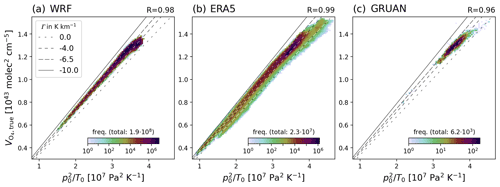Figure 1Density plots of the relation between the O4 VCD and ${p}_{\mathrm{0}}^{\mathrm{2}}/{T}_{\mathrm{0}}$ for (a) WRF data for May to June 2018 from 07:00 to 17:00 UTC, (b) ERA5 data for the 4 selected days in 2018, and (c) all available GRUAN profiles. Frequency per pixel is color coded, with a binning of 100 pixels for both x and y axis, as in all 2D frequency distributions shown below. Respective correlation coefficients are provided in the top right of each panel. Lines display the expected dependency according to Eq. (10) for different lapse rates. Low values correspond to high-altitude sites with low surface pressure; for GRUAN, only few of such stations are available (Boulder and La Réunion at 1.7 and 2.2 km altitude, respectively). The very high values for ERA5 and GRUAN are observed for polar regions with cold temperatures.

${V}_{{\mathrm{O}}_{\mathrm{4}},\phantom{\rule{0.25em}{0ex}}\mathrm{true}}$ varies considerably for all datasets, where the low values are caused by mountains due to reduced pressure, while the very high values for ERA5 and GRUAN are caused by cold temperatures in polar regions. The variability of ${V}_{{\mathrm{O}}_{\mathrm{4}},\phantom{\rule{0.25em}{0ex}}\mathrm{true}}$ is well reflected in ${p}_{\mathrm{0}}^{\mathrm{2}}/{T}_{\mathrm{0}}$, and very good correlation between both quantities is found, with most data points in accordance with plausible lapse rates in the range of −4 to −6.5 K km−1. For the WRF simulations for Germany, most data points match a lapse rate close to −6.5 K km−1. ERA5 and GRUAN data show higher variability in slopes, as they also cover a wider range of atmospheric conditions.

proposed to determine the O4 VCD based on vertical profiles of T and p constructed from the respective surface values by assuming a constant tropospheric lapse rate of −6.5 K km−1. We can use Eq. (10) for the same purpose, but without the need for constructing full vertical profiles. Figures 2 and 3 display maps of the deviation δΓ between parameterized and true O4 VCD for WRF and ERA5, respectively, assuming a constant lapse rate of −6.5 K km−1. Results for additional days for ERA5 are shown in Appendix C.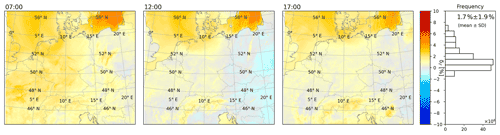Figure 2Deviation δΓ according to Eq. (19) for WRF simulations at 07:00, 12:00, and 17:00 UTC on 1 May 2018. On the right, the frequency distribution of δΓ and its mean and SD are given for the WRF simulation period from May to June 2018.

Generally, good agreement between ${V}_{{\text{O}}_{\mathrm{4}},\phantom{\rule{0.25em}{0ex}}\mathrm{\Gamma }}$ and ${V}_{{\mathrm{O}}_{\mathrm{4}},\phantom{\rule{0.25em}{0ex}}\mathrm{true}}$ is found (Fig. 2): on average, δΓ is 1.7 %; i.e., ${V}_{{\text{O}}_{\mathrm{4}},\phantom{\rule{0.25em}{0ex}}\mathrm{\Gamma }}$ are higher than ${V}_{{\mathrm{O}}_{\mathrm{4}},\phantom{\rule{0.25em}{0ex}}\mathrm{true}}$ by 1.7 %. Over land around noon, δΓ is close to 0. Over ocean, however, δΓ is higher (about 3 % up to 7 %).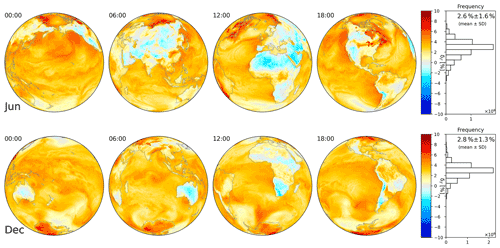Figure 3Deviation δΓ according to Eq. (19) for ERA5 at 00:00, 06:00, 12:00, and 18:00 UTC on 18 June (top) and 18 December (bottom) 2018. The projection focuses on daytime for each time step. On the right, the frequency distribution and mean and SD are given for all hourly outputs of the respective day. Results for 18 March and 18 September 2018 are shown in Fig. C1.

For ERA5 data on 18 June 2018, δΓ over Germany is close to 0 as well (Fig. 3). On a global scale, however, only moderate agreement is found between ${V}_{{\text{O}}_{\mathrm{4}},\phantom{\rule{0.25em}{0ex}}\mathrm{\Gamma }}$ and ${V}_{{\mathrm{O}}_{\mathrm{4}},\phantom{\rule{0.25em}{0ex}}\mathrm{true}}$, with a mean deviation of 2.6 %. High values for δΓ are found generally over ocean. In particular over cold water surfaces, like the west coast of North America and South America, the Hudson Bay, or the Great Lakes, δΓ is very high (up to 7 %). This is related to temperature inversions close to ground: due to the too low surface temperatures (as compared to a polytropic atmosphere with the same O4 VCD), the O4 VCD calculated from Eq. (10) is biased high. This will be discussed in detail in Sect. 5.2. Over continents, δΓ is lower, and generally close to 0, except over deserts, where negative values are observed.

## 4.2 Effective lapse rate and relative humidity at ground

Figure 4 displays the effective lapse rate, as defined in Eq. (9), for ERA5 data from 18 June 2018, clearly showing that the general patterns of systematic deviations seen in Fig. 3 are mainly caused by the simple assumption of a globally constant lapse rate in the calculation of δΓ.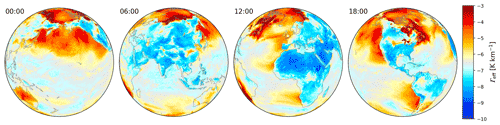Figure 4Effective lapse rate Γeff as defined in Eq. (9) for ERA5 profiles on 18 June 2018.

In Fig. 5a, the effective lapse rate is compared to the actual lapse rate between ground and 5 km altitude above ground, revealing a correlation of 0.83. Figure 5b displays the relation between relative humidity at ground and the effective lapse rate (R=0.59). Assuming a linear relation between Γeff and RH0 in Eq. (13) results in a linear relation between RH0 and the ratio Q (Eq. 18). This is displayed in Fig. 5c. Interestingly, the correlation (R=0.79) is far better than in (b) (R=0.59). This indicates that the parameterization based on RH0 at least partly corrects for other simplifications made in the formalism in Sect. 2.3, in particular the neglect of humidity in the ideal gas law.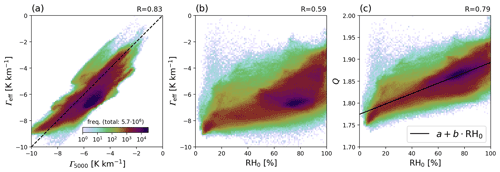Figure 5Relations between lapse rate, RH0, and ratio Q for ERA5 data on 18 June 2018. (a) Relation between the actual lapse rate (calculated from the temperature difference between ground and 5 km altitude) and the effective lapse rate. (b) Relation between RH0 and effective lapse rate. (c) Relation between RH0 and the ratio Q as defined in Eq. (18), where the black line shows the linear fit $a+b\cdot {\text{RH}}_{\mathrm{0}}$.

We use ERA5 data from 18 June 2018 to determine the parameters a and b in Eq. (14) by applying a linear least squares fit to the data presented in Fig. 5c, as shown by the black line. Fitted parameters are $a=\mathrm{1.77434}±\mathrm{0.00003}$ and $b=\mathrm{0.11821}±\mathrm{0.00004}$ (for RH0 in absolute numbers, i.e., 0.5 for 50 % RH). The corresponding parameterization of the effective lapse rate (Eq. 13) is ${\mathrm{\Gamma }}_{\text{eff}}=\left(-\mathrm{7.709}+\mathrm{4.038}\cdot {\text{RH}}_{\mathrm{0}}\right)$ K km−1, which yields −7.709, −5.690 and −3.671 K km−1 for RH0 of 0 %, 50 %, and 100 %, respectively.

## 4.3O4 VCD as function of p0, T0, and RH0

With Eq. (14), an empirical parameterization of the O4 VCD was derived based on surface values of temperature, pressure, and relative humidity. We applied this parameterization to all investigated datasets. Figures 6 and 7 display δRH for WRF and ERA5, respectively. GRUAN results are shown in Fig. 8.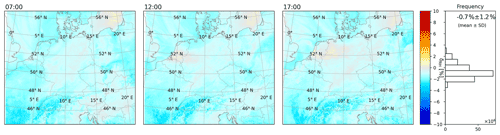Figure 6Deviation δRH according to Eq. (19) for WRF simulations at 07:00, 12:00, and 17:00 UTC on 1 May 2018. On the right, the frequency distribution and mean and SD are given for the WRF simulation period from May to June.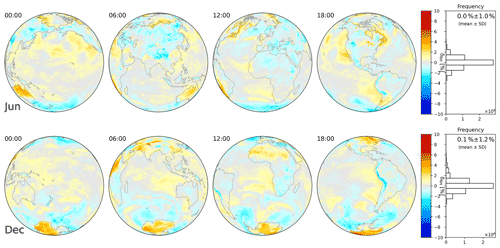Figure 7Deviation δRH according to Eq. (19) for ERA5 at 00:00, 06:00, 12:00, and 18:00 UTC on 18 June (top) and 18 December (bottom) 2018. The projection focuses on daytime for each time step. On the right, the frequency distribution and mean and SD are given for all hourly outputs of the respective day. Note that the mean deviation on 18 June 2018 is 0 by construction, as the parameters a and b (Eq. 14) are optimized for this day. Results for 18 March and 18 September 2018 are shown in Fig. C2.

For the WRF simulations, δΓ was already quite close to 0 (mean δΓ=1.6 %; see Fig. 2). δRH (Fig. 6) is closer to 0, but now showing a slight negative bias (mean ${\mathit{\delta }}_{\text{RH}}=-\mathrm{0.7}$ %). Variability has reduced considerably (SD of δRH is 1.2 %, compared to 1.9 % for δΓ). δRH shows a weaker land–ocean contrast. Over the Alps, δRH is biased low (down to −3 %).

For ERA5, the parameterization involving RH is a substantial improvement compared to the results for δΓ. The large difference between land (in particular deserts) and oceans seen in δΓ (Fig. 3) is strongly reduced for δRH (Fig. 7). For 18 June 2018, the mean of δRH≡0.0 % is of course a consequence of the fit optimizing a and b which is based on the same ERA5 dataset. But also for 18 December 2018, the mean deviation is close to zero. The SD reduces from 1.6 % for δΓ to 1.0 % for δRH on 18 June 2018, and from 1.3 % to 1.2 % on 18 December 2018. Applying Eq. (14) to ECMWF data from other months yields similar results, as shown in Fig. C2, with largest deviations of 0.2±1.8 % observed for 18 March 2018.

Remaining systematic deviations in the maps of δRH are due to

• weather, for instance associated with low pressure or frontal systems. This reflects the simplifying assumptions made, in particular assuming hydrostatic conditions in Sect. 2. Note, however, that MAX-DOAS retrievals are usually not considered for weather conditions associated with rain and clouds.

• cold surfaces causing temperature inversions, as discussed in more detail in Sect. 5.2.

• mountains, which tend to show systematic deviations δRH that are mostly negative (e.g., over the Andes or the Himalayas). For further discussion see Sect. 5.4.

• some patterns of enhanced δRH at the northern, southern, or western edge of the maps for ERA5, corresponding to polar regions as well as sampling times shortly after sunrise (e.g., over southern Africa at 06:00 UTC).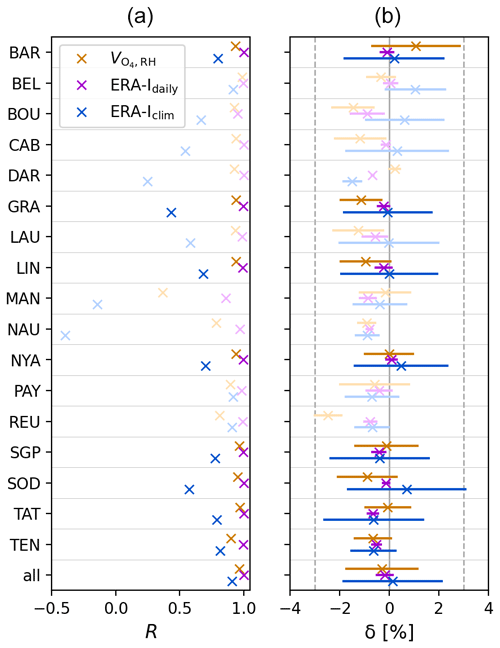Figure 8Comparison to the true O4 VCD in terms of temporal correlation coefficient (a) and deviation δ according to Eq. (19(b) for all GRUAN stations for O4 VCDs calculated from (a) Eq. (14) based on GRUAN surface values (orange), (b) daily ERA-Interim profiles (purple) and (c) profiles from the ERA-Interim climatology (blue), interpolated to the GRUAN measurements in space and time. Light colors indicate stations with fewer than 100 profiles (compare Table B1).

So far, the formalism derived in Sect. 2 has been applied to data from meteorological models. Now we test it for measured profiles from radiosondes as well. Application of Eq. (14) to GRUAN data generally yields deviations close to 0 between parameterized and true O4 VCDs for all stations, as shown in Fig. 8. Parameterized and true VCD show high correlations, indicating that the temporal variability of the atmospheric state is well captured by the simple parameterization based on surface values alone. The mean deviation δRH of all considered GRUAN profiles is −0.3 %, with a SD of 1.4 %. For 11 out of the 17 stations, the mean agreement is within 1 %. Largest deviations are found for La Réunion (REU), where ${V}_{{\text{O}}_{\mathrm{4}},\phantom{\rule{0.25em}{0ex}}\text{RH}}$ is biased low by −2.5 %. This is probably related to the altitude of this station of more than 2 km on a remote island in the Indian Ocean. Highest positive deviation of 1.1 % is found for Utqiaġvik, Alaska (formerly Barrow, which is the name used in the GRUAN dataset), with also highest SD of 1.8 %. This is caused by some very high values during spring where surface temperatures are very low (<240 K) and temperature inversions occur.

5 Discussion

## 5.1 Comparison to existing methods for the calculation of the O4 VCD

Within MAPA , the O4 VCD has been so far determined by integrating vertical profiles of the O4 number density based on full profiles of T, p, and RH, which are by default taken from daily ERA-Interim simulations (ERA-Idaily), or, as fallback solution, from a monthly climatology complied from multi-annual ERA-Interim data (ERA-Iclim), both on 1 resolution.

Thus, we evaluate the performance of the proposed simple calculation of the O4 VCD by comparing the results for GRUAN profiles, where the true O4 VCD is known, also to the ERA-Interim profiles interpolated in space and time. In addition, a correction of surface altitude is necessary: for La Réunion, for instance, the radio sondes were launched at a surface altitude of 2 km, while the surface altitude in ERA-Interim (with 1 resolution) is just 54 m. This could easily cause deviations of 10 % in O4 VCDs when ignored. Thus we apply the following correction to the ERA-Interim profiles:

• In the case of GRUAN station altitude being higher than ERA-Interim surface altitude, the ERA-Interim profiles of T and RH are just linearly interpolated. As pressure profiles are almost exponential, ln (p) is linearly interpolated.

• In the case of GRUAN station altitude being lower than ERA-Interim surface altitude, ERA-Interim profiles are extended by surface values of T0 and ln (p0) as derived from linear extrapolation of T and ln (p), respectively. RH at ground, however, is not extrapolated, as this might result in unphysical values of RH below 0 or above 1. Instead, the value of the lowest ERA-Interim model layer is taken as RH0.

We calculate the deviation from the true VCD (defined by the GRUAN profiles) according to Eq. (19) for daily and climatological ERA-Interim data. The correlation coefficients as well as mean and SD of the resulting deviations are also included in Fig. 8.

For O4 VCDs based on daily ERA-Interim profiles, the agreement to VCDs integrated from GRUAN profiles is generally very good. Correlation coefficients are almost 1, and deviations are close to 0 for most stations. Only for mountainous sites such as Boulder, where surface altitude differs between GRUAN and ERA-Interim, clear deviations from 0 are found.

Results based on the ERA-Interim climatology, however, show far weaker correlation than for daily ERA-Interim data, as they do not resolve day-to-day changes in meteorology. Mean deviations are within ±1 % for most stations, with a SD of about 2 %.

In comparison to these existing methods, the O4 VCDs based on Eq. (14) are worse than those based on daily ERA-Interim profiles but significantly better than those based on a profile climatology, in particular in terms of correlation and SD.

## 5.2 Impact of temperature inversions

The presented parameterizations derive the O4 VCD just from surface values of T, p, and RH. This requires some basic assumptions about the atmospheric profile shape. In the case of temperature inversions, these assumptions do not hold. Thus, we focused on daytime conditions by selecting only data with $\text{SZA}<\mathrm{85}{}^{\circ }$. But still, temperature inversions can also occur during daytime, in particular over cold water and ice surfaces, as well as shortly after sunrise.

Figure 9 displays temperature inversions, here defined as the difference between tropospheric maximum and surface temperature, for ERA5 data on 18 June 2018. Strong temperature inversions are found, e.g., over Hudson Bay or the Great Lakes where sea surface temperature is low. Also at the western edge of the illuminated Earth (i.e., shortly after sunrise), temperature inversions occur, e.g., in northern and southern Africa at 06:00 UTC, indicating remnants of nocturnal profiles.

Large parts of the regions with high positive deviation δΓ (Fig. 3) or δRH (Fig. 7) actually correspond to temperature inversions. Thus, for MAX-DOAS measurements close to cold surface waters or other regions with temperature inversions, the formalism of Eqs. (10) and (14) should only cautiously be applied, and corrections of surface temperature might be needed for better results.

As the impact of temperature inversions on δΓ is quite strong, we skip profiles with temperature inversions of more than 2 K for the investigation of the effects of humidity (Sect. 5.3) and surface altitude (Sect. 5.4) in order to avoid interference of different effects.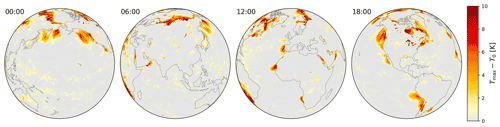Figure 9Temperature inversions, expressed as difference between tropospheric maximum and surface temperature, for ERA5 simulations at 00:00, 06:00, 12:00, and 18:00 UTC on 18 June 2018.

## 5.3 Impact of humidity

The formalism in Sect. 2.3 is assuming dry air. Addition of humidity results in lower O2 and O4 number densities, which significantly affects the O4 VCD, especially in the tropics . Humidity affects all terms in Eq. (6) (i.e., the O2 VCD, the O2 surface number density, and the ratio of effective heights of O2 and O4), but it cannot be accounted for in the formalism without completely losing the simplicity of Eq. (10).

However, these effects are partly accounted for in Eq. (14), with empirically determined parameters a and b, since the ratio Q was determined based on the true O4 VCD where humidity effects were appropriately accounted for.

In order to check for possible remaining impacts of humidity on the performance of Eq. (14), we check how far δRH is related to specific humidity at ground (Fig. 10). In addition, we compare δRH also to the total column water vapor, as this provides information on humidity in the full column, not only at surface. In both cases, correlations are low, and no significant impact of humidity on δRH could be found.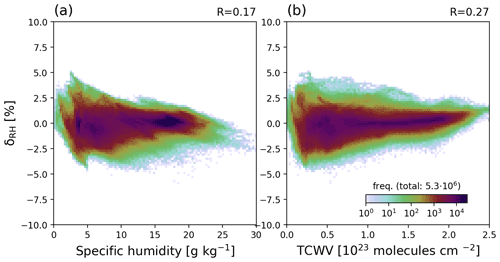Figure 10Deviation δRH according to Eq. (19) as function of (a) specific humidity at ground, and (b) TCWV for ERA5 simulations on 18 June 2018. Temperature inversions with ${T}_{\text{max}}-{T}_{\mathrm{0}}>\mathrm{2}$ K have been skipped.

## 5.4 Impact of surface altitude

Figures 6 and 7 reveal systematic spatial patterns in δRH corresponding to mountains. We thus investigate a possible relation between surface altitude and δRH for all investigated datasets (Fig. 11).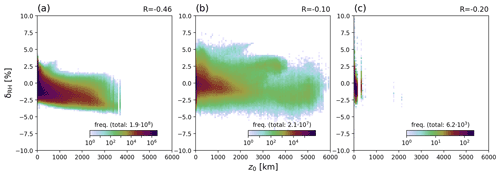Figure 11Dependency of δRH on surface altitude for (a) WRF data for May to June 2018, (b) ERA5 data for all selected days, and (c) GRUAN data. For (b), temperature inversions with ${T}_{\text{max}}-{T}_{\mathrm{0}}>\mathrm{2}$ K have been skipped.

For the WRF simulations, the Alps can be clearly recognized in Fig. 6, with mountains showing lower values of δRH. This can also be seen in the density plot in Fig. 11a, where surface altitude and δRH are anticorrelated with $R=-\mathrm{0.46}$, and a decrease of δRH of roughly 1 % km−1. For GRUAN stations (c), results are similar, but statistics are poor, and the correlation coefficient is low, as only two stations (Boulder and La Réunion) are available with a surface altitude above 1 km.

For ERA5, however, results are not as clear as those for WRF. The correlation coefficient is low, and for altitudes between 2 and 3 km, it looks like δRH is increasing rather than decreasing with altitude. And for very high surface altitudes as found over the Himalaya, δRH is still close to 0 and would not match the slope of 1 % km−1 estimated for WRF.

The reason for the poor correlation between z0 and δRH for ERA5 compared to the WRF results is not clear to us. Obviously, other factors would probably also have to be considered (season, SZA). But since there is no clear correlation, and a quantitative correction would rather worsen δRH instead of improving it for several mountain areas around the globe, we decided not to apply an explicit correction for surface altitude.

Consequently, the parameterization of Eq. (14) has higher uncertainties when applied for mountainous sites: for z0>2 km, δRH is −0.5 % on average with a SD of 1.8 %. But still, the parameterized O4 VCD matches the requirement of accuracy/precision better than 3 % even for elevated sites.

## 5.5 Diurnal cycles

Surface conditions can change rapidly, e.g., in the case of passing frontal systems or storm tracks. For such rapid changes, the change of the true O4 VCD might not be adequately represented by the change of ${V}_{{\text{O}}_{\mathrm{4}},\phantom{\rule{0.25em}{0ex}}\text{RH}}$. These effects are reflected in the SD of deviations δRH for ERA5, WRF, and GRUAN.

In addition, surface values could change systematically during the day in the case of strong solar irradiation, causing a diurnal cycle of surface temperature and the O4 VCD . Thus we investigate the diurnal cycles of T0, p0, RH0, and the respective O4 VCDs ${V}_{{\text{O}}_{\mathrm{4}},\phantom{\rule{0.25em}{0ex}}\text{RH}}$ and ${V}_{{\mathrm{O}}_{\mathrm{4}},\phantom{\rule{0.25em}{0ex}}\mathrm{true}}$ in more detail, and we check how far (a) the WRF simulations reflect the actual diurnal cycles and (b) the parameterized O4 VCDs based on surface values reflect the diurnal cycle of the true O4 VCD. For this, we extract the WRF simulations at the locations of the DWD ground station network. In order to focus on strong diurnal patterns, we select at each station those days where the intra-day change of surface temperature, as recorded by DWD, exceeds 10 K.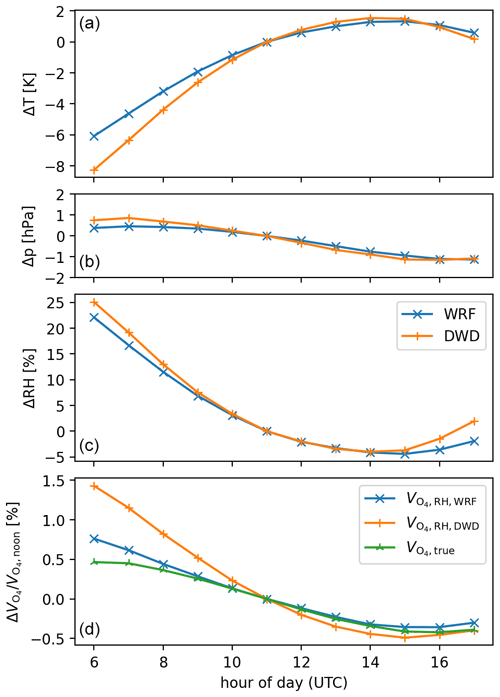Figure 12Diurnal cycles of surface temperature (a), pressure (b), RH (c), and the O4 VCD (d). Data points show the mean values for all stations for May to June 2018 for days where the increase in T0 (from DWD) over the day is larger than 10 K. For better comparison, the mean value at 11:00 UTC (around solar noon for Germany) is subtracted from all datasets. For the O4 VCD, the relative change is shown.

Figure 12 displays the diurnal cycles of surface properties and O4 VCDs for WRF and DWD station data. While surface pressure shows no relevant changes during day, surface temperature increases by 9.8 K from morning to afternoon due to the selection of days with strong diurnal cycle in surface temperature2. For WRF simulations, a similar pattern is found, but the mean temperature increase over the day is smaller (7.4 K). As ${V}_{{\text{O}}_{\mathrm{4}},\phantom{\rule{0.25em}{0ex}}\text{RH}}$ is reciprocal to T0, a change of 10 K in surface temperature alone would correspond to a change of ${V}_{{\text{O}}_{\mathrm{4}},\phantom{\rule{0.25em}{0ex}}\text{RH}}$ of 3.5 %. However, at the same time, RH decreases by about 30 %, which has an opposite effect on ${V}_{{\text{O}}_{\mathrm{4}},\phantom{\rule{0.25em}{0ex}}\text{RH}}$. Consequently, the diurnal cycle of ${V}_{{\text{O}}_{\mathrm{4}},\phantom{\rule{0.25em}{0ex}}\text{RH}}$ is only moderate (about 1.9 % and 1.1 % decrease from morning to evening for DWD and WRF, respectively, where the cycle for WRF is less strong due to the less strong cycle in T0).

The true O4 VCD, as derived from the integrated WRF profiles, also decreases over the day, and agrees well to ${V}_{{\text{O}}_{\mathrm{4}},\phantom{\rule{0.25em}{0ex}}\text{RH}}$ (WRF) in the afternoon. In the morning, however, ${V}_{{\text{O}}_{\mathrm{4}},\phantom{\rule{0.25em}{0ex}}\text{RH}}$ (WRF) is higher compared to noon by 0.8 %, while ${V}_{{\mathrm{O}}_{\mathrm{4}},\phantom{\rule{0.25em}{0ex}}\mathrm{true}}$ is only 0.5 % higher. This deviation between parameterized and true O4 VCD indicates that in the early morning, surface measurements are not as useful for determining the full column, which is probably related to remainders of the nocturnal boundary layer which often has atypical lapse rates due to temperature inversions.

But even during morning hours, the systematic error made by ${V}_{{\text{O}}_{\mathrm{4}},\phantom{\rule{0.25em}{0ex}}\text{RH}}$ is relatively small, at least for the investigated time period for Germany. But also for the global ERA5 analysis, the impact of diurnal cycles on the calculation of the O4 VCD is only moderate; otherwise, Figs. 7 and C2 would show systematic east–west gradients.

Thus, the parameterization of Eq. (14) also reflects most of the diurnal cycle of the O4 VCD, with remaining systematic errors below 0.3 %.

## 5.6 Accuracy and precision

In Eq. (14), we provide a formula for the calculation of the O4 VCD. Accuracy and precision of the resulting ${V}_{{\text{O}}_{\mathrm{4}}}$ thereby depend on accuracy and precision of (1) the chosen parameterization and (2) surface values p0, T0, and RH0.

1. We estimate overall accuracy and precision of Eq. (14) to <1 % and <2 % based on mean and SD of deviations between parameterized and true O4 VCD for WRF, ERA5 and GRUAN data as presented above. Higher deviations can occur in particular in the case of temperature inversions (see Sect. 5.2).

2. Application of Eq. (14) requires surface measurements of p0, T0, and RH0. Uncertainties of temperature and pressure are rather uncritical, as an error of 1 K and 1 hPa for T0 and p0 would correspond to an error of 0.3 % and 0.2 % in ${V}_{{\text{O}}_{\mathrm{4}},\phantom{\rule{0.25em}{0ex}}\text{RH}}$, respectively. In order to reach an accuracy/precision of 1 %, the corresponding errors of RH0 have to be lower than 16 %. These limits should be achievable for adequate meteorological instrumentation and a measurement procedure following World Meteorological Organization (WMO) guidelines. In particular, surface temperature should be measured at about 1.25 to 2 m above ground using a radiation shield (WMO2018).

The proposed parameterization thus allows one to calculate O4 VCD with overall uncertainties below 3 %, which is sufficient for applications in MAX-DOAS profile inversions. Compared to existing methods, the parameterization yields even better results than a profile climatology.

We thus consider the proposed parameterization as a useful approach for determining the O4 VCD for cases where no daily model profiles are available and recommend to also apply it for mountain sites for comparison and possible correction of daily model profiles.

6 Conclusions

The O4 VCD can be expressed in terms of surface pressure and temperature based on physical laws, if a constant lapse rate is assumed, without the need for constructing full vertical profiles. With an empirical correction which parameterizes the effective lapse rate as linear function of surface RH, we could present a formula for simple and quick calculation of the O4 VCD based on p0, T0, and RH0:

$\begin{array}{}\text{(20)}& {V}_{{\text{O}}_{\mathrm{4}},\text{RH}}=\frac{\mathrm{6.733}×{\mathrm{10}}^{\mathrm{39}}}{\mathrm{1.774}+\mathrm{0.1182}\cdot {\text{RH}}_{\mathrm{0}}}\cdot \frac{{p}_{\mathrm{0}}^{\mathrm{2}}}{{T}_{\mathrm{0}}}\phantom{\rule{0.125em}{0ex}}{\mathrm{molecules}}^{\mathrm{2}}\phantom{\rule{0.125em}{0ex}}{\mathrm{cm}}^{-\mathrm{5}}.\end{array}$

This parameterization reproduces the real O4 VCD, as derived from vertically integrated profiles, within $-\mathrm{0.7}±\mathrm{1.2}$ % for WRF simulations around Germany, 0.2±1.8 % for global reanalysis data (ERA5), and $-\mathrm{0.3}±\mathrm{1.4}$ % for radiosonde soundings around the world. Largest deviations are observed in the case of temperature inversions which cause too low T0 (compared to the remaining profile) and thus high biased estimates of ${V}_{{\text{O}}_{\mathrm{4}},\phantom{\rule{0.25em}{0ex}}\text{RH}}$. For applications to measured surface values, uncertainties of 1 K, 1 hPa, and 16 % for temperature, pressure, and RH correspond to relative uncertainties of the O4 VCD of 0.3 %, 0.2 %, and 1 %, respectively.

This accuracy and precision of <3 % is typically lower than other uncertainties of spectral analysis or radiative transfer modeling . Thus, the proposed parameterization is well suited for application in MAX-DOAS profile inversions. Moreover, the parameterization reflects the true O4 VCD, as derived from radiosonde measurements, even better (in particular in terms of temporal correlation and SD) than O4 VCD calculated from a climatology of atmospheric profiles of T, p, and RH. We thus recommend to equip MAX-DOAS measurement stations with a state-of-the-art thermometer (with radiation shield), barometer, and hygrometer.

Appendix A: Ratio of effective heights

The ratio of the effective heights for O4 and O2 in Eq. (6) depends on the shape of the O2 profile. For specific shapes the ratio can be calculated explicitly. Below, we derive the ratio $\frac{{h}_{{\text{O}}_{\mathrm{2}}}}{{h}_{{\text{O}}_{\mathrm{4}}}}$, which allows for simpler notation avoiding compound fractions. For application in Eq. (6), the inverse ratio has to be taken.

## A1 Isothermal atmosphere

For the simple assumption of a barometric pressure profile with constant T, the O2 number density decreases exponentially with altitude:

$\begin{array}{}\text{(A1)}& {n}_{{\text{O}}_{\mathrm{2}}}={n}_{{\text{O}}_{\mathrm{2}},\mathrm{0}}\cdot \mathrm{exp}\left(-{z}^{\prime }/H\right),\end{array}$

with the scale height H. In this case, the integral of Eq. (3) directly yields H; i.e., the effective height equals the scale height for exponential profiles. For O4, the profile is exponentially decreasing as well, with the scale height being half of that for O2. Thus, for O2 profiles declining exponentially with z, the ratio of effective heights is just

$\begin{array}{}\text{(A2)}& \frac{{h}_{{\text{O}}_{\mathrm{2}}}}{{h}_{{\text{O}}_{\mathrm{4}}}}=\mathrm{2}.\end{array}$

## A2 Polytropic atmosphere

If the temperature is changing linearly with altitude, i.e., the dependence of $T\left(z\right)={T}_{\mathrm{0}}+\mathrm{\Gamma }\cdot \left(z-{z}_{\mathrm{0}}\right)$ is described by a constant lapse rate Γ, the resulting profile of O2 follows a power function:

$\begin{array}{}\text{(A3)}& {n}_{{\text{O}}_{\mathrm{2}}}={n}_{{\text{O}}_{\mathrm{2}},\mathrm{0}}\cdot {\left(\mathrm{1}+\frac{\mathrm{\Gamma }}{{T}_{\mathrm{0}}}{z}^{\prime }\right)}^{-\mathit{\alpha }},\end{array}$

with

$\begin{array}{}\text{(A4)}& {z}^{\prime }=z-{z}_{\mathrm{0}}\end{array}$

being altitude above surface, and

$\begin{array}{}\text{(A5)}& \mathit{\alpha }=\mathrm{1}+\frac{g\cdot M}{R\cdot \mathrm{\Gamma }}\end{array}$

being the constant exponent.

Note that for a constant lapse rate, temperature reaches 0 K at an altitude of

$\begin{array}{}\text{(A6)}& {z}_{\text{TOA}}=\frac{{T}_{\mathrm{0}}}{\mathrm{\Gamma }}.\end{array}$

For T0=300 K and $\mathrm{\Gamma }=-\mathrm{6.5}$ K km−1, zTOA is about 46 km.

Thus, Eq. (A3) is defined from ${z}^{\prime }=\mathrm{0}$ to ${z}^{\prime }={z}_{\text{TOA}}$, and ${n}_{{\text{O}}_{\mathrm{2}}}$ is set to 0 above.

Integration of Eq. (3) yields

$\begin{array}{}\text{(A7)}& \begin{array}{rl}{h}_{{\text{O}}_{\mathrm{2}}}& =\underset{\mathrm{0}}{\overset{{z}_{\text{TOA}}}{\int }}{\left(\mathrm{1}+\frac{\mathrm{\Gamma }}{{T}_{\mathrm{0}}}{z}^{\prime }\right)}^{-\mathit{\alpha }}\phantom{\rule{0.25em}{0ex}}\mathrm{d}{z}^{\prime }\\ & ={\left[\frac{\mathrm{1}}{-\mathit{\alpha }+\mathrm{1}}{\left(\mathrm{1}+\frac{\mathrm{\Gamma }}{{T}_{\mathrm{0}}}{z}^{\prime }\right)}^{-\mathit{\alpha }+\mathrm{1}}\cdot \frac{{T}_{\mathrm{0}}}{\mathrm{\Gamma }}\right]}_{\mathrm{0}}^{{z}_{\text{TOA}}}\\ & =\frac{\mathrm{1}}{-\mathit{\alpha }+\mathrm{1}}\cdot \frac{{T}_{\mathrm{0}}}{\mathrm{\Gamma }}.\end{array}\end{array}$

For O4, the number density profile is

$\begin{array}{}\text{(A8)}& {n}_{{\text{O}}_{\mathrm{4}}}={n}_{{\text{O}}_{\mathrm{4}},\mathrm{0}}\cdot {\left(\mathrm{1}+\frac{\mathrm{\Gamma }}{{T}_{\mathrm{0}}}{z}^{\prime }\right)}^{-\mathrm{2}\mathit{\alpha }},\end{array}$

and thus

$\begin{array}{}\text{(A9)}& {h}_{{\text{O}}_{\mathrm{4}}}=\frac{\mathrm{1}}{-\mathrm{2}\mathit{\alpha }+\mathrm{1}}\cdot \frac{{T}_{\mathrm{0}}}{\mathrm{\Gamma }}.\end{array}$

The ratio of effective heights can then be calculated as

$\begin{array}{}\text{(A10)}& \begin{array}{rl}\frac{{h}_{{\text{O}}_{\mathrm{2}}}}{{h}_{{\text{O}}_{\mathrm{4}}}}& =\frac{\mathrm{2}\mathit{\alpha }-\mathrm{1}}{\mathit{\alpha }-\mathrm{1}}\\ & =\frac{\mathrm{2}\frac{g\cdot M}{R\cdot \mathrm{\Gamma }}+\mathrm{1}}{\frac{g\cdot M}{R\cdot \mathrm{\Gamma }}}\\ & =\mathrm{2}+\frac{R}{g\cdot M}\cdot \mathrm{\Gamma }.\end{array}\end{array}$

For a lapse rate of 0 this equals the result for the isothermal atmosphere (≡2). For a typical lapse rate of −6.5 K km−1, the ratio of effective heights is 1.81.

## A3 Impact of the tropopause

In the previous section, the ratio of effective heights was calculated assuming a constant lapse rate throughout the atmosphere. A more realistic approach would be to assume a constant temperature above the tropopause (TP), as was done in . However, with the separation of the atmosphere in troposphere and stratosphere, it would not be possible to express the ratio of effective heights as a simple function of the lapse rate as in Eq. (A10). Thus, we decided to neglect the impact of the tropopause on the ratio of effective heights in the derivation of Eq. (10).

This causes a bias of ${V}_{{\text{O}}_{\mathrm{4}},\phantom{\rule{0.25em}{0ex}}\mathrm{\Gamma }}$ that can be easily quantified from Eq. (10) itself (applied at the tropopause instead of ground): the stratospheric O4 column for constant T is $\frac{C}{\mathrm{2}}\cdot \frac{{p}_{\text{TP}}^{\mathrm{2}}}{{T}_{\text{TP}}}$, while it is $\frac{C}{\mathrm{2}+\frac{R}{g\cdot M}\mathrm{\Gamma }}\cdot \frac{{p}_{\text{TP}}^{\mathrm{2}}}{{T}_{\text{TP}}}$ for constant lapse rate. The difference is 6×1040 molecules2 cm−5 (for TTP=200 K, pTP=193 hPa), which is about 0.45 % of the total O4 VCD.

Thus, the O4 VCD derived from Eq. (10) is higher than the respective VCD resulting from the profile construction proposed in . For ${V}_{{\text{O}}_{\mathrm{4}},\phantom{\rule{0.25em}{0ex}}\text{RH}}$ (Eq. 14), this bias is eliminated by the empirical fit of the parameters a and b to the true O4 VCD.

## A4 Side note: determining the effective lapse rate from direct sun measurements of O2 and O4

The O4 VCD depends on the ratio of effective heights for O2 and O4 (Eq. 6), which can be expressed by the atmospheric lapse rate (Eq. A10). This formalism might also be used in the other direction: from total column measurements of O2 and O4, an effective atmospheric lapse rate can be derived.

$\begin{array}{}\text{(A11)}& \begin{array}{rl}\mathrm{2}+\frac{R}{g\cdot M}\cdot \mathrm{\Gamma }& \phantom{\rule{0.25em}{0ex}}\stackrel{\left(\mathrm{Eq}.\phantom{\rule{0.25em}{0ex}}A\mathrm{10}\right)}{=}\frac{{h}_{{\text{O}}_{\mathrm{2}}}}{{h}_{{\text{O}}_{\mathrm{4}}}}\stackrel{\left(\mathrm{Eq}.\phantom{\rule{0.25em}{0ex}}\mathrm{6}\right)}{=}\frac{{V}_{{\text{O}}_{\mathrm{2}}}}{{V}_{{\text{O}}_{\mathrm{4}}}}\cdot {n}_{{\text{O}}_{\mathrm{2}},\mathrm{0}}\\ & \phantom{\rule{0.25em}{0ex}}\stackrel{\left(\mathrm{Eq}.\phantom{\rule{0.25em}{0ex}}\mathrm{8}\right)}{=}\frac{{V}_{{\text{O}}_{\mathrm{2}}}}{{V}_{{\text{O}}_{\mathrm{4}}}}\cdot \frac{{\mathit{\nu }}_{{\text{O}}_{\mathrm{2}}}\cdot {p}_{\mathrm{0}}}{R\cdot {T}_{\mathrm{0}}},\end{array}\end{array}$

and thus

$\begin{array}{}\text{(A12)}& \mathrm{\Gamma }=\left(\frac{{V}_{{\text{O}}_{\mathrm{2}}}}{{V}_{{\text{O}}_{\mathrm{4}}}}\cdot \frac{{\mathit{\nu }}_{{\text{O}}_{\mathrm{2}}}\cdot {p}_{\mathrm{0}}}{R\cdot {T}_{\mathrm{0}}}-\mathrm{2}\right)\cdot \frac{g\cdot M}{R}.\end{array}$

This formalism might be applied to direct sun measurements, where light paths are well defined by the SZA. Even for limited accuracy of column measurements of O2 and O4, this would allow one to derive time series of an effective lapse rate, reflecting the state of the lower atmosphere.

Appendix B: Datasets

## B1 Regional model and surface measurements

### B1.1 WRF simulations

A nested domain centered at 49.12 N, 10.20 E was set up in Lambert conformal conic (LCC) projection with coarser domain (d01) at 15×15 km2 horizontal resolution and finer domain (d02) at 3×3 km2 resolution (Fig. B1). The spatial extent of the d01 domain is 4800×3416 km2 while that for d02 is 1578×1473 km2. Vertically, the model extends from surface until 50 hPa with 42 terrain following layers in between. For constraining the meteorological initial and lateral boundary conditions, we use the ERA5 reanalysis dataset with a horizontal resolution of $\mathrm{0.25}{}^{\circ }×\mathrm{0.25}{}^{\circ }$ and a temporal resolution of 3 h, downloaded at pressure levels and at the surface. The soil classification, terrain height, and land use patterns were taken from the 21 category Noah-modified IGBP-MODIS land use data.

The model simulations were set up for May and June in 2018. The selection of data with $\text{SZA}<\mathrm{85}{}^{\circ }$ results in a daily coverage from 06:00 to 17:00 UTC. Here we focus on model profiles in the d02 domain. The partial column of O4 above 50 hPa is considered accordingly in the calculation of the true O4 VCD (see Sect. 2.4).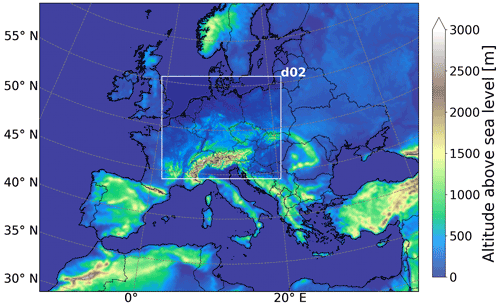Figure B1Nested model domains d01 (full figure) and d02 (marked panel) of the WRF simulations.

### B1.2 DWD weather stations

Germany's National Meteorological Service (Deutscher Wetterdienst, DWD) provides hourly measurements of surface temperature, pressure, and relative humidity for a network of ground stations in Germany . Data are provided via the climate data center web interface (CDC-v2.1; https://cdc.dwd.de/portal/, last access: 18 May 2021). The meteorological measurements are performed in accordance to the guidelines of the WMO to minimize local effects. Additionally, we have applied quality control filters such that the QUALITAETS_BYTE (QB) is below 4 (thereby excluding untested, objected, and calculated values), and QUALITAETS_NIVEAU (QN) is either 3 (automatic control and correction) or 7 (second control done, before correction) to only retain measurements of high quality. By applying these criteria, we retained 98.2 %, 100 %, and 99.5 % of T0, p0, and RH0 data, respectively. Note that using only data with QN =10 (the best possible quality check level) would result in no data left for the period considered in this study. If only QN =7 had been applied, we would have retained the same number of T0 and RH0 but no p0 data.

For this study, we extract DWD measurements for May to June 2018, 06:00 to 17:00 UTC, and only consider stations providing T0, p0, and RH0 simultaneously, resulting in 206 stations which are displayed in Fig. B2.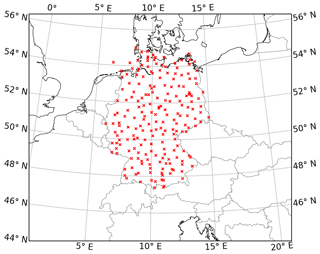Figure B2Location of the 206 DWD ground stations providing simultaneous measurements of surface values of T, p, and RH during May to June 2018.

### B1.3 Validation of WRF surface values

We use the DWD network of surface stations for investigating the accuracy and precision of the WRF simulations. Figure B3 displays correlations between surface values from the DWD station network and the respective WRF simulations. For this purpose, each station is associated with the nearest neighbor from the WRF simulation. We do not interpolate the WRF data as we still want to compare the parameterized O4 VCD with the true VCD derived from vertical integration of the WRF profiles.

Surface altitude (a) is lower in the gridded elevation map used as input in the WRF simulations by 20 m on average, and by almost 1 km for the station on Germany's highest mountain, Zugspitze. This is a consequence of the spatial resolution of the WRF simulations of 1 km, which is not sufficient for resolving single mountains. The systematic negative bias of WRF surface altitude indicates that the DWD stations tend to be located on hill and mountain tops.

This difference in altitude would directly affect the comparisons of T and particularly p. Thus, we apply a simple correction of station values and extrapolate them to the respective WRF surface altitude assuming a lapse rate of −6.5 K km−1. For RH, no correction is applied.

The comparison reveals a good agreement between surface values from WRF and DWD, with remaining systematic biases of WRF simulations of −1 K for T0 and 1 % for RH0.

Table B1List of GRUAN stations and number of available sonde flights (only considering $\text{SZA}<\mathrm{85}{}^{\circ }$) used in this study.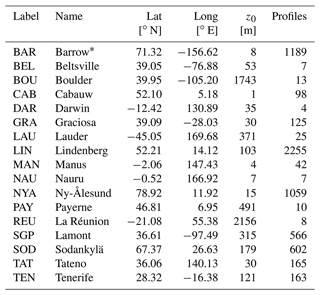* now Utqiaġvik.

## B2 GRUAN stations

The GRUAN stations used in this study are listed in Table B1, including station shortcut and full name, latitude, longitude, altitude of the station, and the number of available profiles with $\text{SZA}<\mathrm{85}{}^{\circ }$. Figure B4 displays a map showing the GRUAN station locations.

The temporal cover of radio sonde measurements at the different stations is displayed in Fig. B5. Note that some stations only contribute a low number of measurements. Still, we decided to keep all stations, as the application of a threshold for a minimum number of profiles of, e.g., 50 would remove all tropical sites (Darwin, Manus, and Nauru).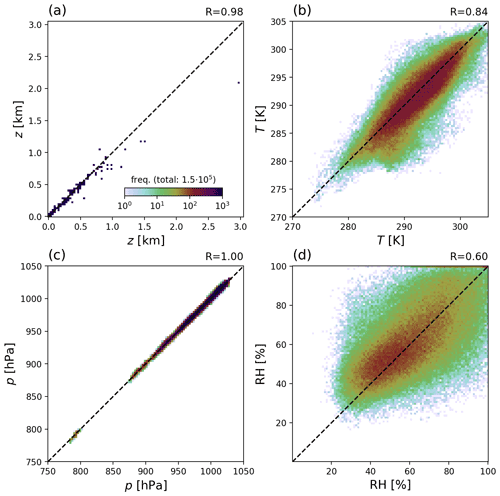Figure B3Comparison of WRF surface values (y axis) to DWD ground stations (x axis). For T and p, station values are adjusted to the mean altitude of the respective gridded elevation map used as input for WRF simulations (see text for details).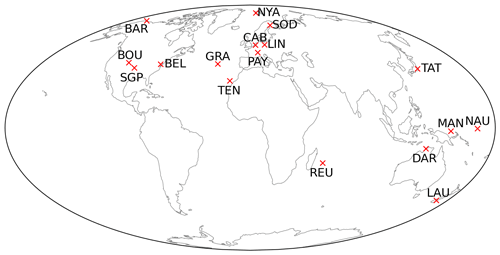Figure B4Location of GRUAN stations considered in this study. For station names and further details see Table B1.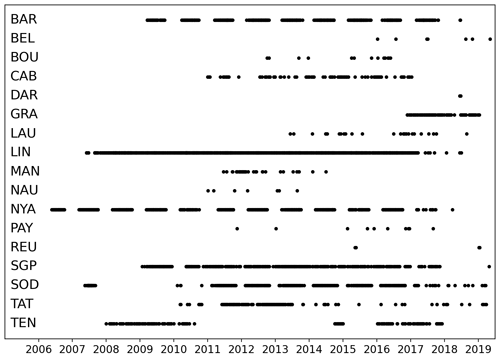Figure B5Time of the available sonde flights (with $\text{SZA}<\mathrm{85}{}^{\circ }$) for the GRUAN stations considered in this study. For station names and further details see Table B1.

Figures C1 and C2 display additional results for δΓ and δRH, respectively, for 18 March and 18 September 2018.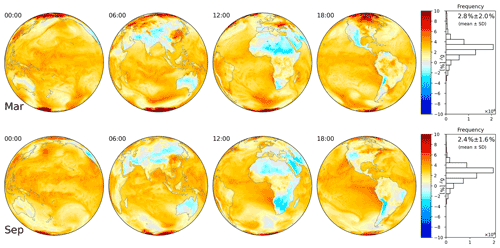Figure C1Deviation δΓ according to Eq. (19) for ERA5 at 00:00, 06:00, 12:00, and 18:00 UTC on 18 March (top) and 18 September (bottom) 2018. The projection focuses on daytime for each time step. On the right, the frequency distribution and mean and SD are given for all hourly outputs of the respective day.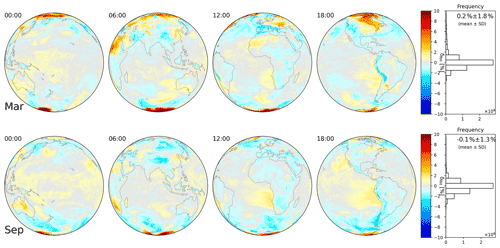Figure C2Deviation δRH according to Eq. (19) for ERA5 at 00:00, 06:00, 12:00, and 18:00 UTC on 18 March (top) and 18 September (bottom) 2018. The projection focuses on daytime for each time step. On the right, the frequency distribution and mean and SD are given for all hourly outputs of the respective day.

Code availability

A Python implementation of the derived functions for the calculation of the O4 VCD is provided in the Supplement.

Data availability

ERA5 data are provided by the European Centre for Medium-Range (, https://doi.org/10.24381/cds.adbb2d47).

Radiosonde measurements were provided by the GRUAN network (; https://doi.org/10.5676/GRUAN/RS92-GDP.2).

Meteorological data for ground stations in Germany were provided by via the climate data center web interface (CDCv2.1; https://cdc.dwd.de/portal/, last access: 18 May 2021).

Supplement

Author contributions

CB initiated this study by proposing to express the O4 VCD by surface number density and column density of O2. VK performed the WRF simulations and preprocessed the DWD data. SD processed ECMWF data. SD, CB, and TW provided input on O4 VCD calculation and meteorology. SB developed the full formalism, performed the intercomparisons to external datasets, and wrote the manuscript, with input and feedback from all co-authors.

Competing interests

At least one of the (co-)authors is a member of the editorial board of Atmospheric Measurement Techniques. The peer-review process was guided by an independent editor, and the authors also have no other competing interests to declare.

Disclaimer

Publisher’s note: Copernicus Publications remains neutral with regard to jurisdictional claims in published maps and institutional affiliations.

Acknowledgements

We would like to thank Rajesh Kumar (UCAR Boulder) and Sergey Osipov and Andrea Pozzer (both MPIC Mainz) for support in setting up the WRF simulations. We thank MPCDF Garching for providing computation resources for the WRF simulations. ERA Interim and ERA5 data used in this study are provided by the European Centre for Medium-Range Weather Forecasts (ECMWF). Meteorological data for ground stations in Germany were provided by Deutscher Wetterdienst (German Weather Service, DWD). Radiosonde measurements were provided by the GRUAN network.

Financial support

The article processing charges for this open-access publication were covered by the Max Planck Society.

Review statement

This paper was edited by Michel Van Roozendael and reviewed by two anonymous referees.

References

Acarreta, J. R., de Haan, J. F., and Stammes, P.: Cloud pressure retrieval using the O2-O2 absorption band at 477 nm, J. Geophys. Res., 109, D05204, https://doi.org/10.1029/2003JD003915, 2004. a

Beirle, S., Dörner, S., Donner, S., Remmers, J., Wang, Y., and Wagner, T.: The Mainz profile algorithm (MAPA), Atmos. Meas. Tech., 12, 1785–1806, https://doi.org/10.5194/amt-12-1785-2019, 2019. a, b

Bodeker, G. E., Bojinski, S., Cimini, D., Dirksen, R. J., Haeffelin, M., Hannigan, J. W., Hurst, D. F., Leblanc, T., Madonna, F., Maturilli, M., Mikalsen, A. C., Philipona, R., Reale, T., Seidel, D. J., Tan, D. G. H., Thorne, P. W., Vömel, H., and Wang, J.: Reference Upper-Air Observations for Climate: From Concept to Reality, B. Am. Meteorol. Soc., 97, 123–135, https://doi.org/10.1175/BAMS-D-14-00072.1, 2016. a

Deutscher Wetterdienst: CDCv2.1, Climate Data Center (CDC) [data set], https://cdc.dwd.de/portal/, last access: 18 May 2021. a

Dirksen, R. J., Sommer, M., Immler, F. J., Hurst, D. F., Kivi, R., and Vömel, H.: Reference quality upper-air measurements: GRUAN data processing for the Vaisala RS92 radiosonde, Atmos. Meas. Tech., 7, 4463–4490, https://doi.org/10.5194/amt-7-4463-2014, 2014. a

Greenblatt, G. D., Orlando, J., Burkholder, J. B., and Ravishankara, A. R.: Absorption measurements of oxygen between 330 and 1140 nm, J. Geophys. Res., 95, 18577–18582, 1990. a, b

Heckel, A., Richter, A., Tarsu, T., Wittrock, F., Hak, C., Pundt, I., Junkermann, W., and Burrows, J. P.: MAX-DOAS measurements of formaldehyde in the Po-Valley, Atmos. Chem. Phys., 5, 909–918, https://doi.org/10.5194/acp-5-909-2005, 2005. a

Hersbach, H., Bell, B., Berrisford, P., Biavati, G., Horányi, A., Muñoz Sabater, J., Nicolas, J., Peubey, C., Radu, R., Rozum, I., Schepers, D., Simmons, A., Soci, C., Dee, D., and Thépaut, J.-N.: ERA5 hourly data on single levels from 1979 to present. Copernicus Climate Change Service (C3S) Climate Data Store (CDS) [data set], https://doi.org/10.24381/cds.adbb2d47, 2018. a

Hersbach, H., Bell, B., Berrisford, P., Hirahara, S., Horányi, A., Muñoz-Sabater, J., Nicolas, J., Peubey, C., Radu, R., Schepers, D., Simmons, A., Soci, C., Abdalla, S., Abellan, X., Balsamo, G., Bechtold, P., Biavati, G., Bidlot, J., Bonavita, M., Chiara, G. D., Dahlgren, P., Dee, D., Diamantakis, M., Dragani, R., Flemming, J., Forbes, R., Fuentes, M., Geer, A., Haimberger, L., Healy, S., Hogan, R. J., Hólm, E., Janisková, M., Keeley, S., Laloyaux, P., Lopez, P., Lupu, C., Radnoti, G., Rosnay, P. de, Rozum, I., Vamborg, F., Villaume, S., and Thépaut, J.-N.: The ERA5 global reanalysis, Q. J. Roy. Meteor. Soc., 146, 1999–2049, https://doi.org/10.1002/qj.3803, 2020. a

Kaspar, F., Müller-Westermeier, G., Penda, E., Mächel, H., Zimmermann, K., Kaiser-Weiss, A., and Deutschländer, T.: Monitoring of climate change in Germany – data, products and services of Germany's National Climate Data Centre, Adv. Sci. Res., 10, 99–106, https://doi.org/10.5194/asr-10-99-2013, 2013. a

Kreher, K., Van Roozendael, M., Hendrick, F., Apituley, A., Dimitropoulou, E., Frieß, U., Richter, A., Wagner, T., Lampel, J., Abuhassan, N., Ang, L., Anguas, M., Bais, A., Benavent, N., Bösch, T., Bognar, K., Borovski, A., Bruchkouski, I., Cede, A., Chan, K. L., Donner, S., Drosoglou, T., Fayt, C., Finkenzeller, H., Garcia-Nieto, D., Gielen, C., Gómez-Martín, L., Hao, N., Henzing, B., Herman, J. R., Hermans, C., Hoque, S., Irie, H., Jin, J., Johnston, P., Khayyam Butt, J., Khokhar, F., Koenig, T. K., Kuhn, J., Kumar, V., Liu, C., Ma, J., Merlaud, A., Mishra, A. K., Müller, M., Navarro-Comas, M., Ostendorf, M., Pazmino, A., Peters, E., Pinardi, G., Pinharanda, M., Piters, A., Platt, U., Postylyakov, O., Prados-Roman, C., Puentedura, O., Querel, R., Saiz-Lopez, A., Schönhardt, A., Schreier, S. F., Seyler, A., Sinha, V., Spinei, E., Strong, K., Tack, F., Tian, X., Tiefengraber, M., Tirpitz, J.-L., van Gent, J., Volkamer, R., Vrekoussis, M., Wang, S., Wang, Z., Wenig, M., Wittrock, F., Xie, P. H., Xu, J., Yela, M., Zhang, C., and Zhao, X.: Intercomparison of NO2, O4, O3 and HCHO slant column measurements by MAX-DOAS and zenith-sky UV–visible spectrometers during CINDI-2, Atmos. Meas. Tech., 13, 2169–2208, https://doi.org/10.5194/amt-13-2169-2020, 2020. a

Lawrence, M. G.: The Relationship between Relative Humidity and the Dewpoint Temperature in Moist Air: A Simple Conversion and Applications, B. Am. Meteorol. Soc., 86, 225–234, https://doi.org/10.1175/BAMS-86-2-225, 2005. a

May, R. M., Arms, S. C., Marsh, P., Bruning, E., Leeman, J. R., Goebbert, K., Thielen, J. E., and Bruick, Z.: MetPy: A Python Package for Meteorological Data [computer software], https://doi.org/10.5065/D6WW7G29, 2021 (code available at: https://github.com/Unidata/MetPy, last access: 9 February 2022). a

Platt, U. and Stutz, J.: Differential Optical Absorption Spectroscopy, Springer-Verlag Berlin Heidelberg, https://doi.org/10.1007/978-3-540-75776-4, 2008. a

Romps, D. M.: Exact Expression for the Lifting Condensation Level, J. Atmos. Sci., 74, 3891–3900, https://doi.org/10.1175/JAS-D-17-0102.1, 2017. a

Skamarock, W. C., Klemp, J. B., Dudhia, J., Gill, D. O., Liu, Z., Berner, J., Wang, W., Powers, J. G., Duda, M. G., Barker, D. M., and Huang, X.-Y.: A Description of the Advanced Research WRF Model Version 4, UCAR/NCAR, https://doi.org/10.5065/1DFH-6P97, 2019. a

Sommer, M., Dirksen, R., and Immler, F.: RS92 GRUAN Data Product Version 2 (RS92-GDP.2), GRUAN Lead Centre [data set], https://doi.org/10.5676/GRUAN/RS92-GDP.2, 2012.  a, b

Thalman, R. and Volkamer, R.: Temperature dependent absorption cross-sections of O2–O2 collision pairs between 340 and 630 nm and at atmospherically relevant pressure, Phys. Chem. Chem. Phys., 15, 15371–15381, https://doi.org/10.1039/C3CP50968K, 2013. a, b

Tohjima, Y., Machida, T., Watai, T., Akama, I., Amari, T., and Moriwaki, Y.: Preparation of gravimetric standards for measurements of atmospheric oxygen and reevaluation of atmospheric oxygen concentration, J. Geophys. Res., 110, D11302, https://doi.org/10.1029/2004JD005595, 2005. a

Veefkind, J. P., de Haan, J. F., Sneep, M., and Levelt, P. F.: Improvements to the OMI O2–O2 operational cloud algorithm and comparisons with ground-based radar–lidar observations, Atmos. Meas. Tech., 9, 6035–6049, https://doi.org/10.5194/amt-9-6035-2016, 2016. a

Wagner, T., Erle, F., Marquard, L., Otten, C., Pfeilsticker, K., Senne, T., Stutz, J., and Platt, U.: Cloudy sky optical paths as derived from differential optical absorption spectroscopy observations, J. Geophys. Res., 103, 25307–25321, https://doi.org/10.1029/98JD01021, 1998. a

Wagner, T., Beirle, S., Benavent, N., Bösch, T., Chan, K. L., Donner, S., Dörner, S., Fayt, C., Frieß, U., García-Nieto, D., Gielen, C., González-Bartolome, D., Gomez, L., Hendrick, F., Henzing, B., Jin, J. L., Lampel, J., Ma, J., Mies, K., Navarro, M., Peters, E., Pinardi, G., Puentedura, O., Puķīte, J., Remmers, J., Richter, A., Saiz-Lopez, A., Shaiganfar, R., Sihler, H., Van Roozendael, M., Wang, Y., and Yela, M.: Is a scaling factor required to obtain closure between measured and modelled atmospheric O4 absorptions? An assessment of uncertainties of measurements and radiative transfer simulations for 2 selected days during the MAD-CAT campaign, Atmos. Meas. Tech., 12, 2745–2817, https://doi.org/10.5194/amt-12-2745-2019, 2019. a, b, c, d, e, f, g, h, i, j, k

Wagner, T., Dörner, S., Beirle, S., Donner, S., and Kinne, S.: Quantitative comparison of measured and simulated O4 absorptions for one day with extremely low aerosol load over the tropical Atlantic, Atmos. Meas. Tech., 14, 3871–3893, https://doi.org/10.5194/amt-14-3871-2021, 2021. a

World Meteorological Organization (WMO): Guide to Instruments and Methods of Observation, Volume I: Measurement of Meteorological Variables, 2018 Edition, WMO, Geneva, ISBN 978-92-63-10008-5, available at: https://library.wmo.int/index.php?lvl=notice_display&id=12407#.YO1QPEyxXYQ (last access: 13 July 2021), 2018. a

Note that, for this approach, as well as for the parameterizations presented in this study, temperature inversions are problematic. As MAX-DOAS applications require daylight, however, nighttime inversion layers are irrelevant for this study. The remaining temperature inversions at daytime, mostly occurring in early morning hours and over cold water and ice surfaces, will be discussed in Sect. 5.2.

Note that the mean change is lower than the threshold used for the selection of DWD stations. This is caused by averaging diurnal cycles with maxima occurring at different times of the day.